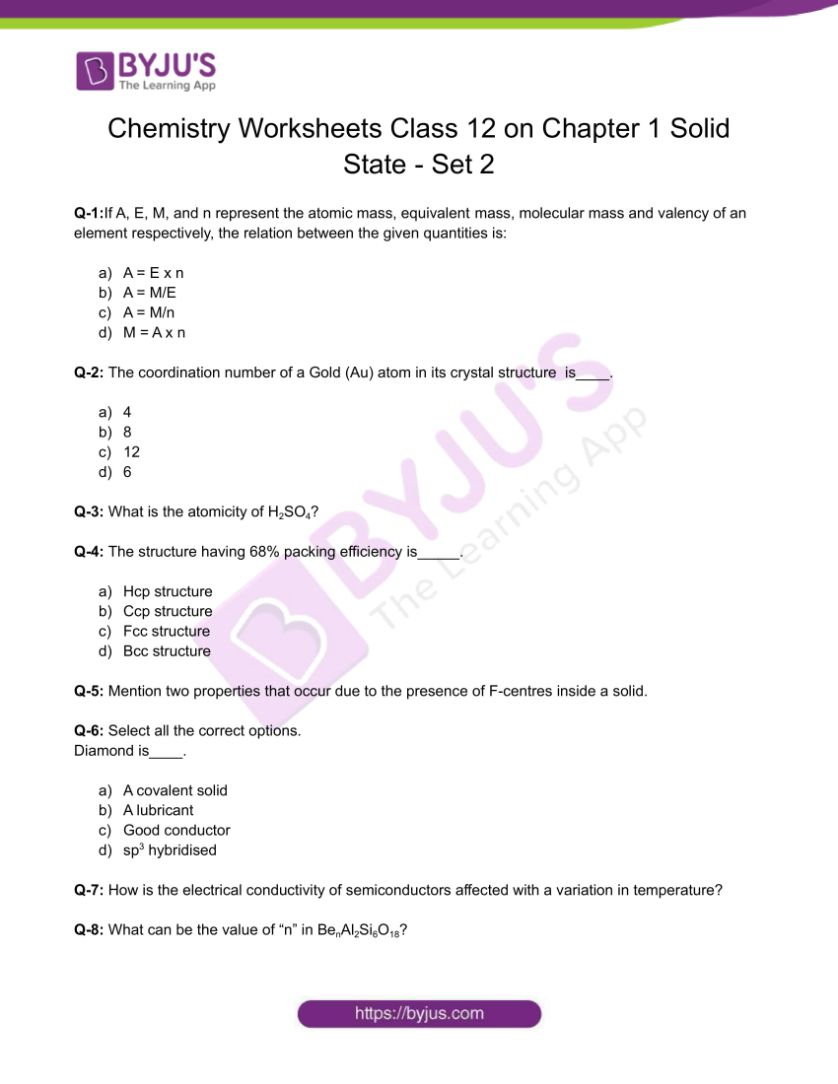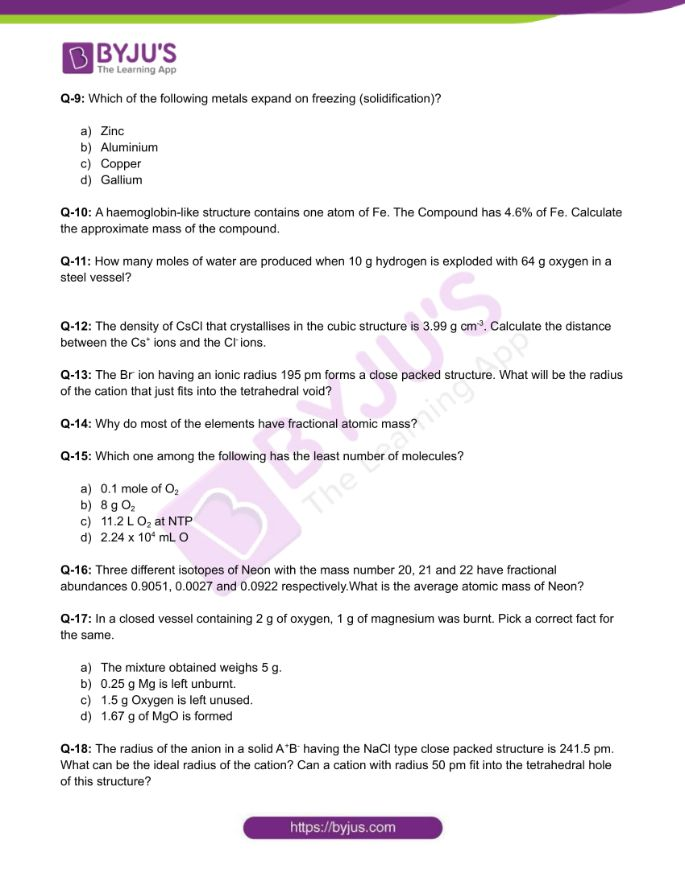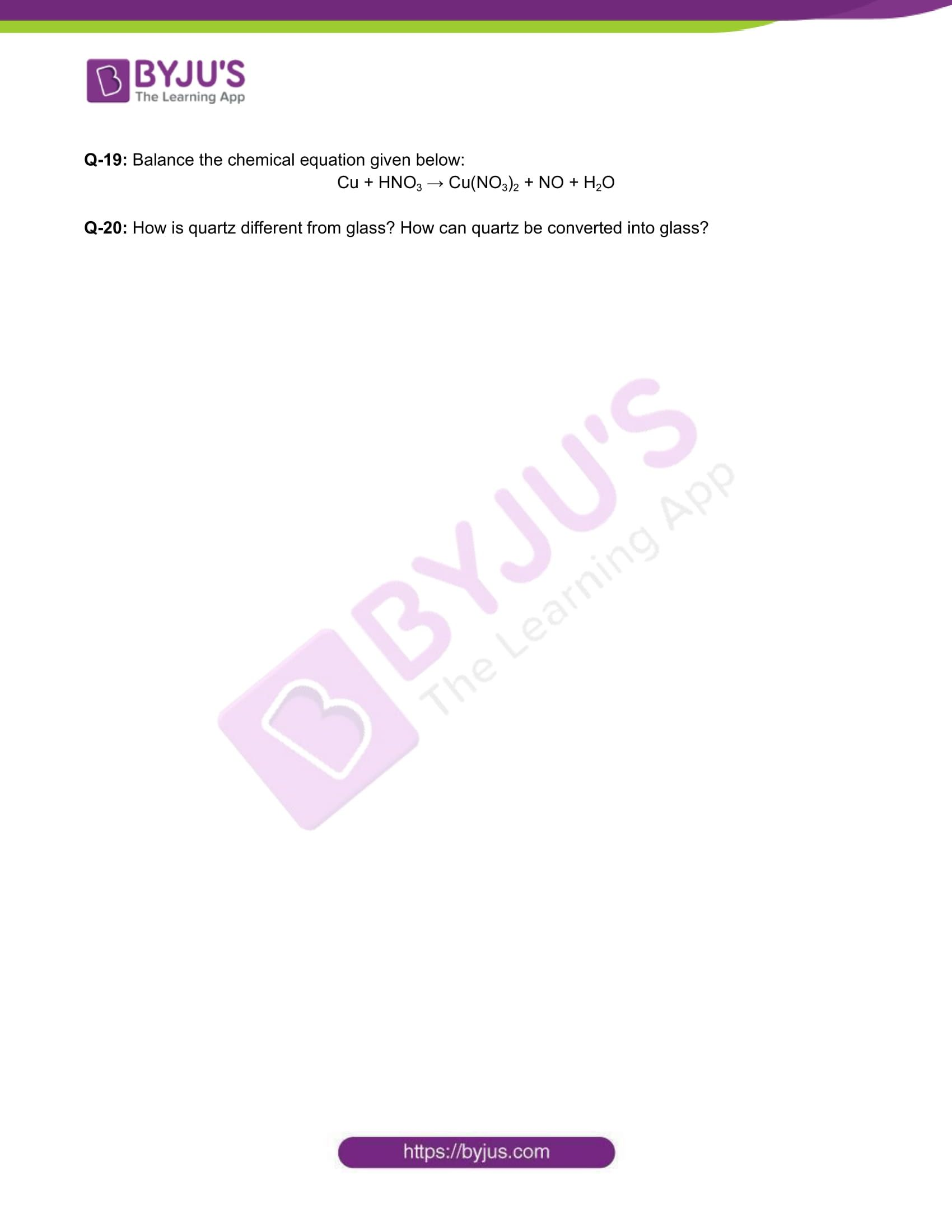Checkout JEE MAINS 2022 Question Paper Analysis : Checkout JEE MAINS 2022 Question Paper Analysis :

# Chemistry Worksheets Class 12 on Chapter 1 Solid State with Answers - Set 2

Solid state materials are most commonly found in our surroundings. The solid state particles are rigid and do not possess fluidity. The particles of solids are bonded together by strong intermolecular forces of attraction due to which the particles do not move to and fro from their mean positions. Solids possess definite shape and definite volume.

## 👇## CBSE Class 12 Chemistry Chapter 1 Solid State Worksheet – Set 2

Q-1:If A, E, M, and n represent the atomic mass, equivalent mass, molecular mass and valency of an element respectively, the relation between the given quantities is:

1. A = E x n
2. A = M/E
3. A = M/n
4. M = A x n

Q-2: The coordination number of a Gold (Au) atom in its crystal structure is____.

1. 4
2. 8
3. 12
4. 6

Q-3: What is the atomicity of H2SO4?

Q-4: The structure having 68% packing efficiency is_____.

1. Hcp structure
2. Ccp structure
3. Fcc structure
4. Bcc structure

Q-5: Mention two properties that occur due to the presence of F-centres inside a solid.

Q-6: Select all the correct options.

Diamond is____.

1. A covalent solid
2. A lubricant
3. Good conductor
4. sp3 hybridised

Q-7: How is the electrical conductivity of semiconductors affected with a variation in temperature?

Q-8: What can be the value of “n” in BenAl2Si6O18?

Q-9: Which of the following metals expand on freezing (solidification)?

1. Zinc
2. Aluminium
3. Copper
4. Gallium

Q-10: A haemoglobin-like structure contains one atom of Fe. The Compound has 4.6% of Fe. Calculate the approximate mass of the compound.

Q-11: How many moles of water are produced when 10 g hydrogen is exploded with 64 g oxygen in a steel vessel?

Q-12: The density of CsCl that crystallises in the cubic structure is 3.99 g cm-3. Calculate the distance between the Cs+ ions and the Clions.

Q-13: The Br ion having an ionic radius 195 pm forms a close packed structure. What will be the radius of the cation that just fits into the tetrahedral void?

Q-14: Why do most of the elements have fractional atomic mass?

Q-15: Which one among the following has the least number of molecules?

1. 0.1 mole of O2
2. 8 g O2
3. 11.2 L O2 at NTP
4. 2.24 x 104 mL O2

Q-16: Three different isotopes of Neon with the mass number 20, 21 and 22 have fractional abundances 0.9051, 0.0027 and 0.0922 respectively.What is the average atomic mass of Neon?

Q-17: In a closed vessel containing 2 g of oxygen, 1 g of magnesium was burnt. Pick a correct fact for the same.

1. The mixture obtained weighs 5 g.
2. 0.25 g Mg is left unburnt.
3. 1.5 g Oxygen is left unused.
4. 1.67 g of MgO is formed

Q-18: The radius of the anion in a solid A+B having the NaCl type close packed structure is 241.5 pm. What can be the ideal radius of the cation? Can a cation with radius 50 pm fit into the tetrahedral hole of this structure?

Q-19: Balance the chemical equation given below:

Cu + HNO3 → Cu(NO3)2 + NO + H2O

Q-20: How is quartz different from glass? How can quartz be converted into glass?

Download the PDF to access answers to the Chemistry Worksheet for Class 12 Chemistry Chapter 1 Solid State Set -2.